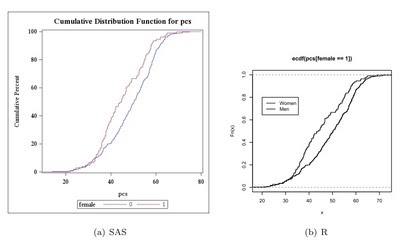## Saturday, August 1, 2009

### Example 7.8: Plot two empirical cumulative density functions using available tools

The empirical cumulative density function (CDF) (section 5.1.16) is a useful way to compare distributions between populations. The Kolmogorov-Smirnov (section 2.4.2) statistic D is the value of x with the maximum distance between the two curves. As an example, we compare the male and female distributions of pcs from the HELP data set described in the book. Here, we use built-in tools to plot the graph; in later entries we will build it from scratch for greater control.

We begin by reading in the data (section 1.1.14) as a comma separated file from the book web site (section 1.1.6).

SAS
`filename myurl   url 'http://www.math.smith.edu/sasr/datasets/help.csv'   lrecl=704;proc import   datafile=myurl out=ds dbms=dlm;   delimiter=',';   getnames=yes;run;`

SAS proc univariate can do this plot automatically (section 5.1.15). It is designed to compare two groups within the data set, using the class statement (section 3.1.3).

`proc univariate data=ds;   var pcs;   class female;   cdfplot pcs / overlay;run;`

In R, the plot() function accepts ecdf() objects (section 5.1.15) as input. Applying this to pcs, conditional on including only the rows when female is 1 (section B.4.2) creates the first empirical CDF as well as the axes. The lines() function (section 5.2.1) also accepts ecdf() objects as input, and applying this to pcs when female is 0 adds the second empirical CDF to the existing plot. A legend (section 5.2.14) is added to show which curve is which. (Note that the Blogger software prevents displaying this image large enough to see the difference here, but it will be visible when run locally.

R
`> ds <- read.csv(   "http://www.math.smith.edu/sasr/datasets/helpmiss.csv")> attach(ds)> plot(ecdf(pcs[female==1]), verticals=TRUE, pch=46)> lines(ecdf(pcs[female==0]), verticals=TRUE, pch=46)> legend(20, 0.8, legend=c("Women", "Men"), lwd=1:3)`

Click the graphic below for a more legible image of the output.Anonymous said...

thanks for the R plot!

Anonymous said...

Thanks! it was really useful, I wonder if you could help me, I need to create a table with data from that cumulative curve,how could I make it?
thanks!

Nick Horton said...

The knots() function is helpful here. Try:

res = ecdf(pcs[female==1])
knots(res)
cbind(knots(res), res(knots(res)))

Unknown said...

data titfig;
set titers;
where tit=1;
run;

proc univariate data=titfig;
var ln10Post;
class armname; ****A vs B ****;
cdfplot ln10Post / overlay;
run;

How to create the reverse Cumulative dist curve....